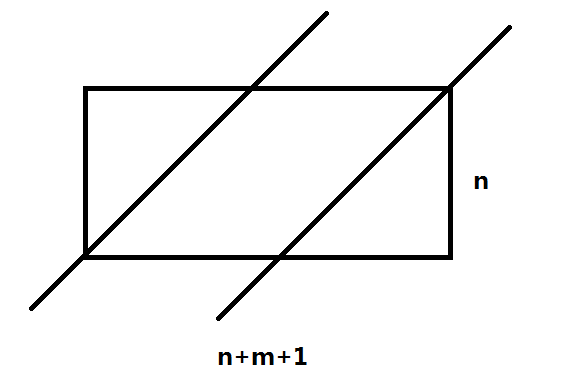# JLOI2015试题大意及部分题解

667 篇文章 1 订阅

================Day1===============

T1:求 (b+d2)n $(\frac{b+\sqrt d}2)^n$的整数部分对p取模后的值

TMD我居然看错题了= = 我居然没有看到【整数部分】这四个字= =

T2:给定一棵树，每个节点是一座城池，有一个坚固程度 hi $h_i$，和两个参数 ai $a_i$ vi $v_i$，其中 ai{0,1} $a_i\in\{0,1\}$
m $m$个骑士，每个骑士有一个初始攻击力xi$x_i$，从某个节点出发，沿该节点向父亲节点一路攻克

18357写了个Splay启发式合并调了3h然后爆零。。。
wyfcyx写了倍增不知道为什么也爆零了。。。
【wyfcyx：等代码发下来之后你们看看我代码就知道怎么回事了】
= =

T3：给定 n $n$个武器，每个武器有m$m$个属性和一个价格，如果一个武器的属性可以被其它几个武器线性表示，那么B君就不会购买这个武器，求最多买多少武器以及买最多武器的前提下最少花多少钱
n,m500 $n,m\leq500$

================Day2===============
T1:求有多少个 n $n$m$m$列的矩阵满足每个数都在 [0,m] $[0,m]$之间且 ai,j<ai,j+1,ai,j<ai1,j+1 $a_{i,j}……很不幸我只会处理 nm $n\leq m$的情况= = n>m $n>m$的情况貌似很复杂？

UPD:扒了一下午毕姥爷的标程之后终于看懂怎么做了QAQ

(官方题解中写的 Cn2n+m $C_{n+m}^{n-2}$是不对的！~)

T2:给定一张 n1000,m3000 $n\leq1000,m\leq3000$的图，每条边有边权，有 p10 $p\leq10$个关键点，每个关键点有一个颜色，现在要求一个边权和最小的生成子图，使同种颜色的关键点互相连通

fS $f_S$表示颜色 S $\in S$的所有点构成的最小斯坦纳生成森林(其中 S $S$是颜色的集合)

fS=max{fS,fT+fST|TS}$f_S=\max\{f_S,f_T+f_{S-T}|T\subset S\}$

3p+C(p2,p21)3p2+C(p2,p22)3p4+...+C(p2,0)30 $3^p+C(\frac p2,\frac p2-1)*3^{p-2}+C(\frac p2,\frac p2-2)*3^{p-4}+...+C(\frac p2,0)*3^0$
=10p2 $=10^{\frac p2}$

UPD：我傻逼吧一遍斯坦纳树就能搞出所有颜色集合的最小斯坦纳树了为何要做 2p2 $2^{\frac p2}$遍= =

(我写的不好只拿到80分

T3:给定一棵满二叉树，每个点有AB两种状态，你可以任意指定，若某个节点和子树中的某个叶节点同时选择A状态会产生一个贡献，若同时选择B状态会产生另一个贡献，要求选择A状态的人不超过 m $m$个，求贡献总和最大

AC爷就是屌

Day2就这样130滚粗了= = 然后我居然Rank2(3)?
18357凭借第三题的Accepted怒拿Rank1 跪跪跪跪跪

10-1862
12-2611010-1677
01-1513
12-0533
08-0313
01-1440
01-28290
01-31150
04-02291
05-15107
04-16509
02-27151
07-1748
01-03135
08-27238
02-01920
02-02567
09-28101点击重新获取扫码支付余额充值